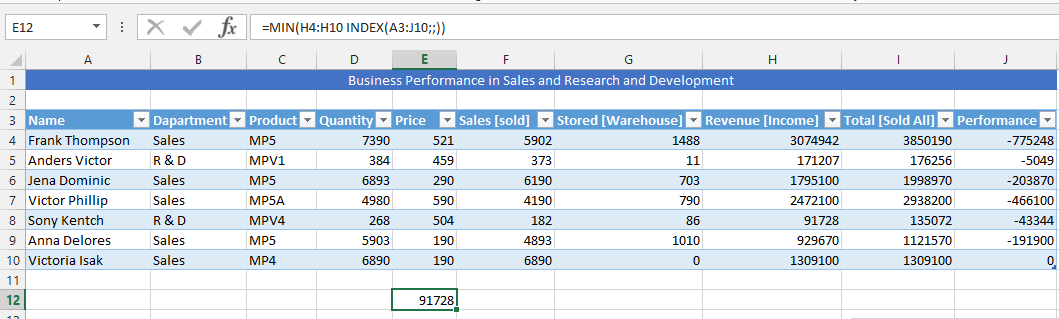#### How to Use Index Function in Excel

An index is a function in the Microsoft Excel with two different forms of syntax: reference and array. When using it in the array form, then it will be returning with either a value or array of different values. Array form is useful when first argument is constant to array.

## Index Formula and Parameters (Arguments)

The index function has different arguments or parameters as some people calls it. The complete formula is array, then row number (row_num), followed by column number (column_num), which is the last part of the index’s arguments.

1. Array: This is a range of either cells or constant array. If the array has one row/column, then a row number or column number that corresponds to it is optional. If an array has more than one row/column to used, and only either a column or row number is used, then the index will return in the array of a whole column or row that is in the array.
2. Row number (row_num): This will select a row that is in the array, from which it can return to with its value. If this is omitted, then it is critical to include the column number. It is important that the argument point to a cell that is inside the array, so it does not return with the #REF! value, which is an error.
3. Column number (column_num): The user has the opportunity to select a column that is in an array, from which it can return with a value. If this is omitted, then the row number is essential to the index function formula. This is an argument that must be pointing to a cell that is within an array, otherwise it will be returning with the #REF error value.

## Examples of the Index Function

Let’s explore practical examples of how to use the Index function in Excel:

### Simple Index Formula

Imagine you want to evaluate the sales performance of an employee, specifically Frank Thompson. The Index function can help you find the number of sales he has made.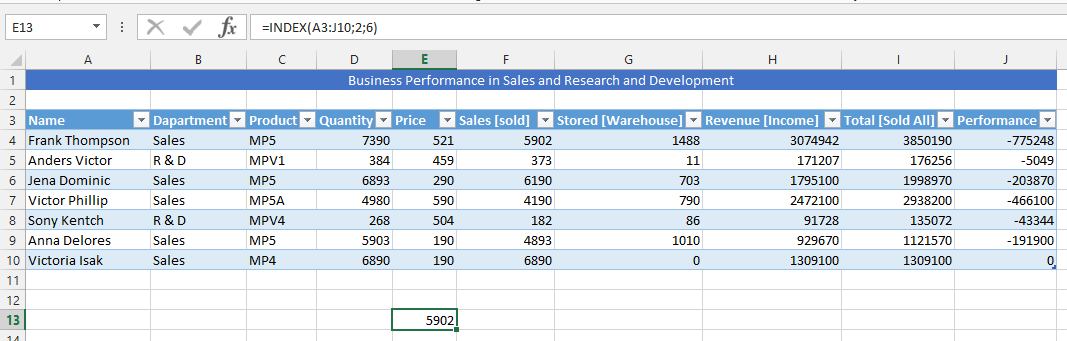### Formula with Named Table

Suppose you have a named table called “Table1,” and you want to extract specific data. This example demonstrates how to use the Index function with a named table.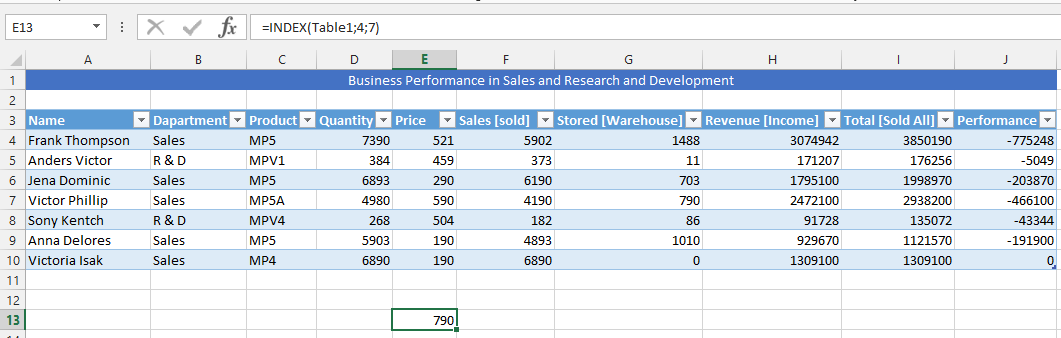### Index and Average Formula

For inventory management, you need to find the average of stored products’ values.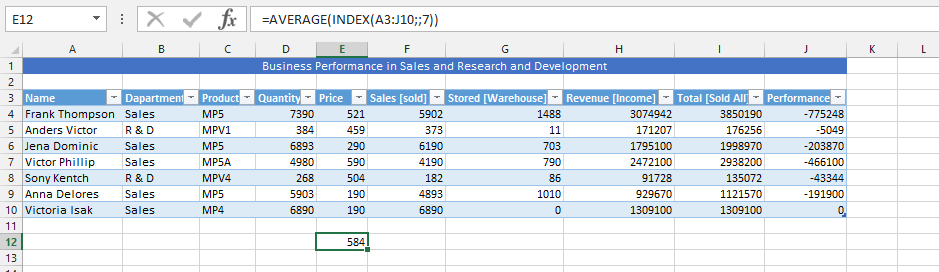### Using the Index and VLOOKUP

To determine the value of stored inventory (products) when sold, you can use both Index and VLOOKUP functions.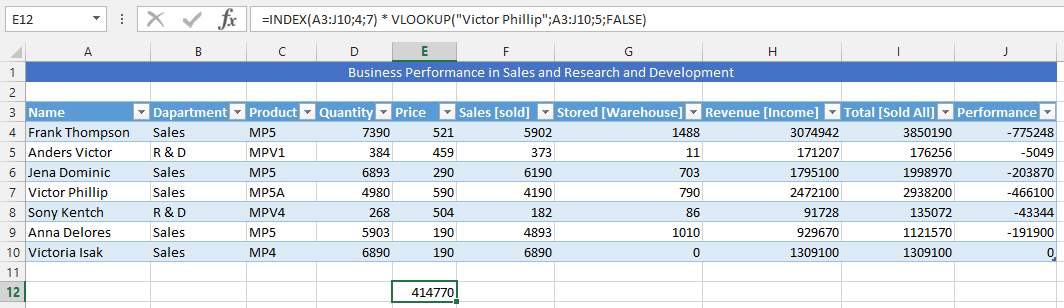### Simultaneous Use of Sum and Index Formulas

To identify the top-performing person in terms of sales, earnings, and areas of improvement, you can calculate the total quantity of products stored in the warehouse.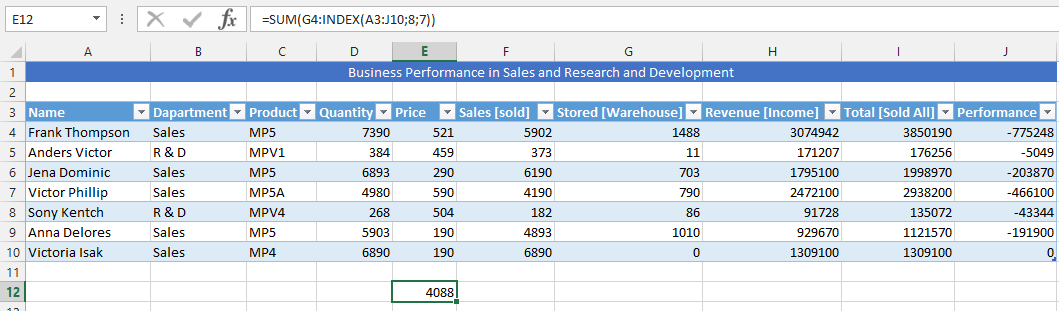### COUNTA with Index Formula

This example utilizes both the Index and COUNTA functions to evaluate the performance of a specific period.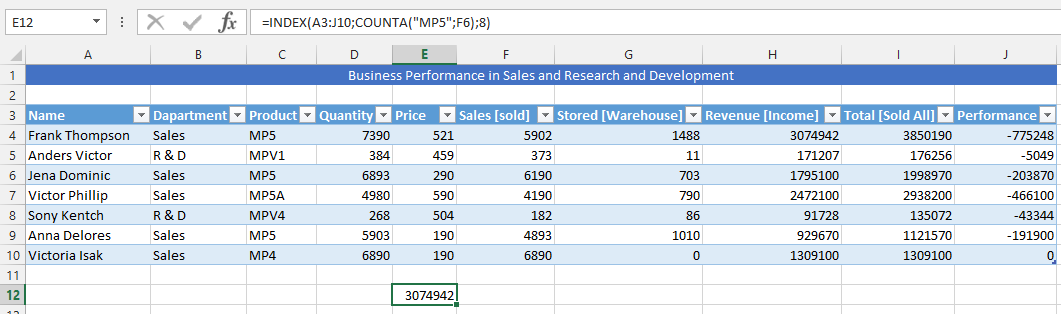### Two Different Index Formula in One

You can use two Index functions within a single formula to multiply values and assess overall business performance.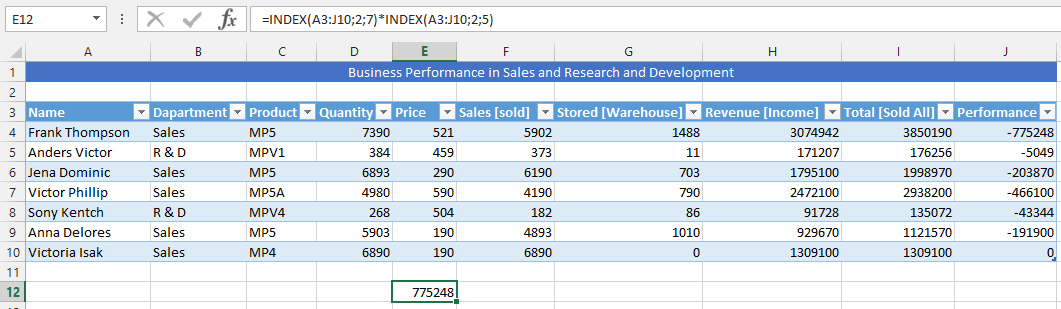### Triple Index Formula

For evaluating financial performance, especially identifying profits and losses, you can use a triple Index formula.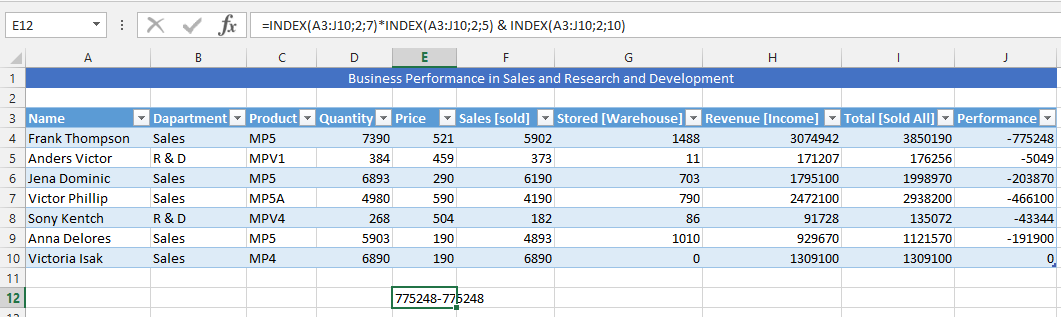### Combining Index and Max Formulas

To identify the highest financial performance and revenue earned in a specific area, you can combine the Index and Max functions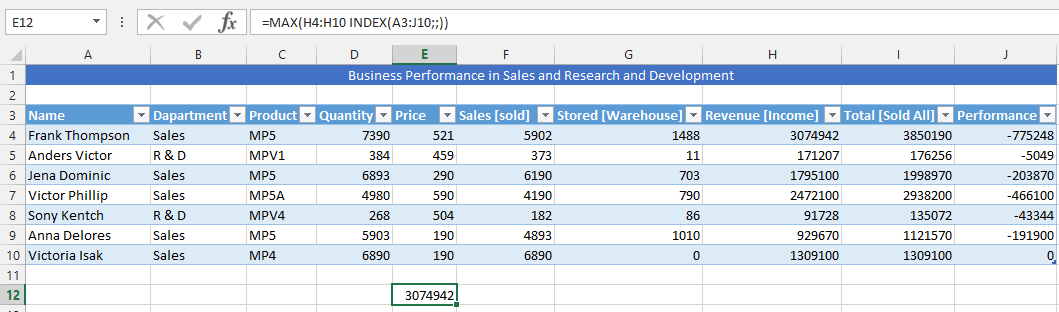### Min and Index Formula in One

Similar to the previous example, you can determine the lowest financial performance and revenue earned using a combination of the Index and Min functions.